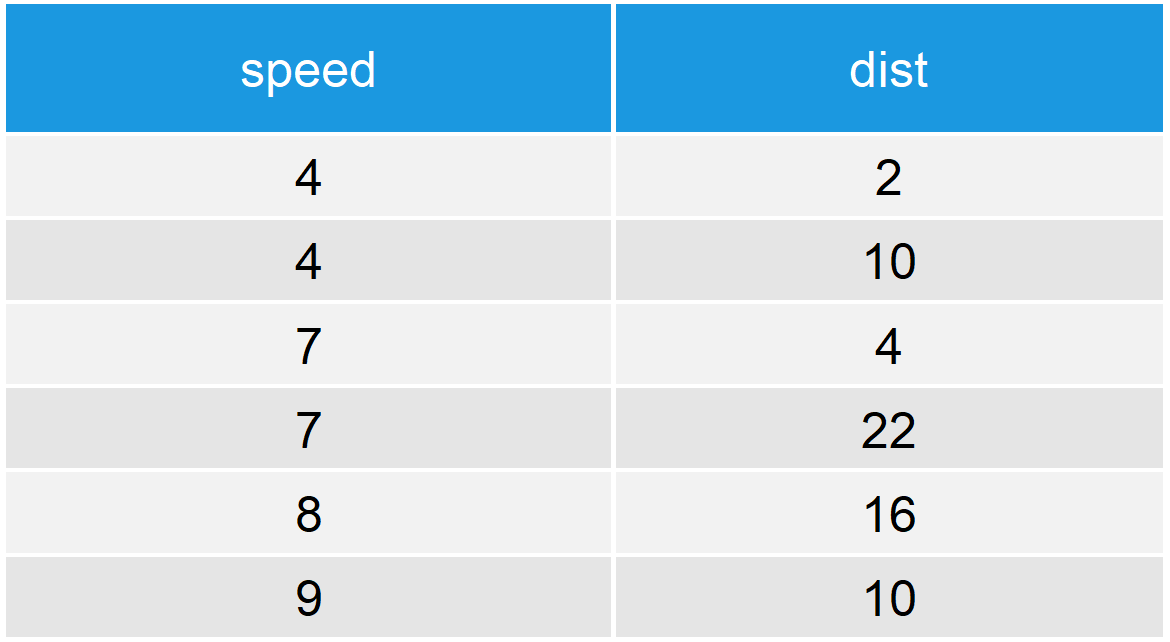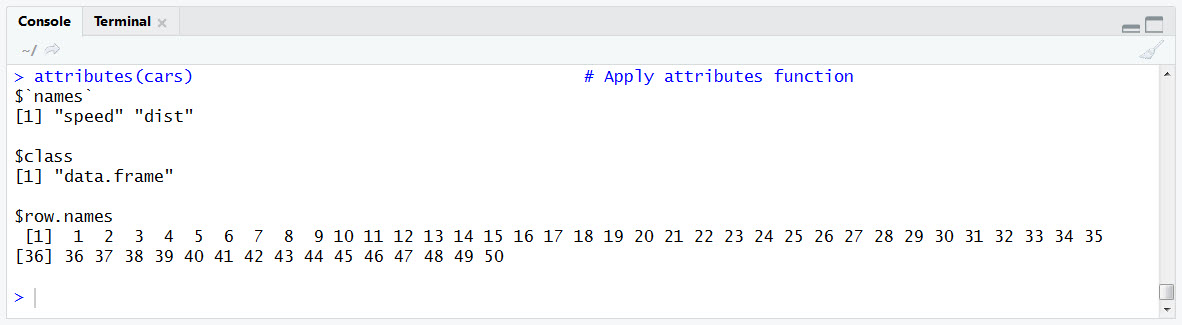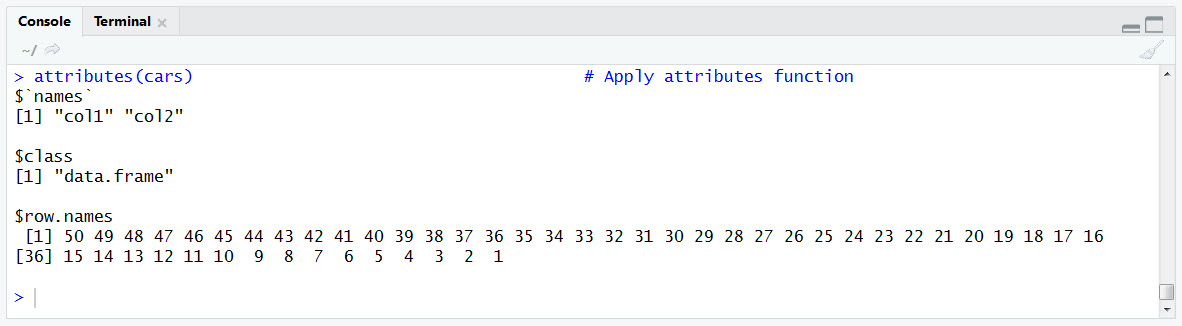# attr, attributes & structure Functions in R | 4 Examples (get, remove & set)

In the following R tutorial, I’m going to show you three functions, with which you can get or set the attributes of your data:

1. attributes function (Examples 1 & 2)
2. attr function (Examples 1 & 3)
3. structure function (Example 4)

The basic R syntax of these three functions looks as follows…

Basic R Syntax:

```attributes(data)

attr(x = data, which = "attribute_name")

structure(data, dim = c(2, 6))```

…and the functions can be defined as follows:

Definitions:

The attributes function returns or sets all attributes of a data object.

The attr function returns or sets one specific attribute of a data object.

The structure function sets additional attributes of a data object.

In the following examples, I’m going to show you the usage of the three functions in more detail. Let’s dive right in!

## Example 1: Basic Application of attr() & attributes()

Let’s begin with a simple example of attr and attributes in R. I’m going to use the cars data set for the example, which can be loaded in R (or RStudio) with the following code:

```data(cars)                                             # Load cars data set
head(cars)                                             # Print first 6 rows of data```Table 1: First 6 Rows of Cars Data Set.

Our example data contains the two numeric columns speed and dist.

Now, let’s apply attributes and attr to this data object:

`attributes(cars)                                       # Apply attributes function`Figure 1: Output of attributes() R Function.

When we apply the attributes R function, all attributes of the car data set are returned as a list (i.e. names, class, and row.names).

So, let’s see what happens when we use the attr function instead:

`attr(x = cars, which = "names")                        # Apply attr function`Figure 2: Output of attr() R Function.

As you can see, with the attr function we can access and extract a single attribute of our data (i.e. names).

Why? Because attr requires a precise specification, which attributes should be printed (and we chose to return the names attribute).

In summary: The main difference between attributes() and attr() is that attributes returns everything, while attr returns only selected values.

So how can we use this in practice? That’s what I’m going to show you in Examples 2 and 3…

## Example 2: Assign New Attributes with the attributes() R Function

In Example 2, I’ll show you how to change all attributes of a data frame. For this task, we first need to define a list that contains all new attributes…

```attributes_list <- list(names = c("col1", "col2"),     # Set different column names
class = "data.frame",          # Retain data.frame class
row.names = nrow(cars):1)      # Reverse order of row.names```

…and then we can use attributes() in order to remove the old attributes and set these new attributes:

`attributes(cars) <- attributes_list                    # Set all attributes of data`

Let’s print the updated attributes to the RStudio console:

`attributes(cars)                                       # Apply attributes function`Figure 3: Output of attributes() R Function After Updating All Attributes.

As you can see, we changed the names attribute to “col1” and “col2”, we kept the data.frame class, and we reversed the order of the row.names.

Easy! So let’s move on to attr()…

## Example 3: Change One Attribute via attr()

As you have seen in Example 1, with the R attr command we can specify the attributes that we want to replace. This can make the R syntax much simpler, in case you need to change only one out of many attributes:

`attr(x = cars, which = "names") <- c("aaa", "bbb")     # Set only names attribute`

In only one line of code, we replaced the names attribute:

`attributes(cars)                                       # Apply attributes function`Figure 4: Output of attributes() R Function After Updating Only One Attribute.

Much simpler than in Example 2!

Stay with me, in the next example I’m going to show you another useful function for the manipulation of attributes…

## Example 4: The R structure Function

A related R command to attributes() and attr() is the structure() function.

In which situations can it be helpful? Let me show with an example…

First, let’s create an example vector in R:

```set.seed(12345)                                        # Set seed for reproducibility
vec <- round(runif(15, 0, 10))                         # Create one-dimensional vector
vec                                                    # Print vector to console
# 7  9  8  9  5  2  3  5  7 10  0  2  7  0  4```

As you can see, we have created a simple vector with 15 numeric values.

In order to change the shape of this vector, we can use the structure R function:

```vec_dim <- structure(vec, dim = c(3, 5))               # Change dimension of vector
vec_dim                                                # Print updated vector
#      [,1] [,2] [,3] [,4] [,5]
# [1,]    7    9    3   10    7
# [2,]    9    5    5    0    0
# [3,]    8    2    7    2    4```

As you can see, we have manipulated the dimension attribute via dim = c(3, 5).

In other words: The structure function is useful when you want to add additional attributes to a data object!

## Video: Objects & Attributes in R

You want to learn even more about objects and attributes in R? Then I can recommend the following YouTube video of Roger Peng.

Please accept YouTube cookies to play this video. By accepting you will be accessing content from YouTube, a service provided by an external third party.If you accept this notice, your choice will be saved and the page will refresh.

Subscribe to the Statistics Globe Newsletter

•January 26, 2019 7:08 am

Very useful tutorials. Thanks a lot, Sir.

•January 26, 2019 7:36 am

Thank you Shrinivas, nice to hear!

•